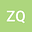Singular limit of Lam\’{e} equations
•## Abstract

In this paper, we study the asymptotic behavior of the solution to the Lam\’{e} equations with a parameter $\var$. We prove that the solution will converge to the solution of a Maxwell type equations as $\var\rightarrow0$; Meanwhile we will show that the solution converges to the solution of a Stokes type equations as $\var\rightarrow\infty$.
02 Jul 2021Submitted to Mathematical Methods in the Applied Sciences
05 Jul 2021Submission Checks Completed
05 Jul 2021Assigned to Editor
10 Jul 2021Reviewer(s) Assigned
20 Nov 2021Review(s) Completed, Editorial Evaluation Pending
11 Nov 2022Editorial Decision: Revise Minor